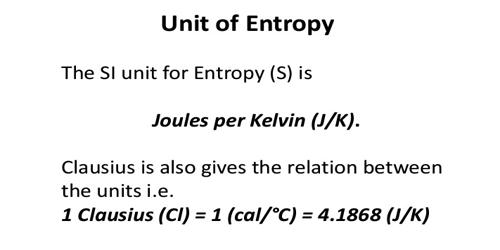Chemistry

# How to Measure Units of Entropy?Units of entropy: Entropy is defined as the quantitative measure of disorder or randomness in a system. The dimension of entropy is energy in terms of heat X temperature-1. The entropy is expressed as calories per degree which are referred to as the entropy units (eu). Since entropy also depends on the quantity of the substance, the unit of entropy is calories per degree per mole (or) eu. per mole. The temperature in this equation must be measured on the absolute, or Kelvin temperature scale. At absolute 0 (0 K), all atomic motion ceases and the disorder in a substance is zero.

cgs units of entropy are cal.K-1 denoted as eu. The SI unit is JK-1 and denoted EU. 1 eu = 4.184 EU.

viii) Entropy change is related to enthalpy change as follows:

For a reversible and isothermal process,

∆Srev = ∆qrev/T

Since ΔH is the heat absorbed (or) evolved in the process at constant T and pressure P.ΔS is also calculated

from ΔH as ΔS = ΔH/T

where T is the temperature of the process involving ΔH, amount of enthalpy change, at constant pressure.

Entropy is considered to be an extensive property of matter that is expressed in terms of energy divided by temperature. The SI units of entropy are J/K (joules/degrees Kelvin).

Measurement: In an isothermal process, the change in entropy (delta-S) is the change in heat (Q) divided by the absolute temperature (T):

delta-S = Q/T

In any reversible thermodynamic process, it can be represented in calculus as the integral from a process’s initial state to its final state of dQ/T. In a more general sense, entropy is a measure of probability and the molecular disorder of a macroscopic system. In a system that can be described by variables, those variables may assume a certain number of configurations. If each configuration is equally probable, then the entropy is the natural logarithm of the number of configurations, multiplied by Boltzmann’s constant:

S = kB ln W

where S is entropy, kB is Boltzmann’s constant, ln is the natural logarithm, and W represents the number of possible states. Boltzmann’s constant is equal to 1.38065 × 10−23 J/K.